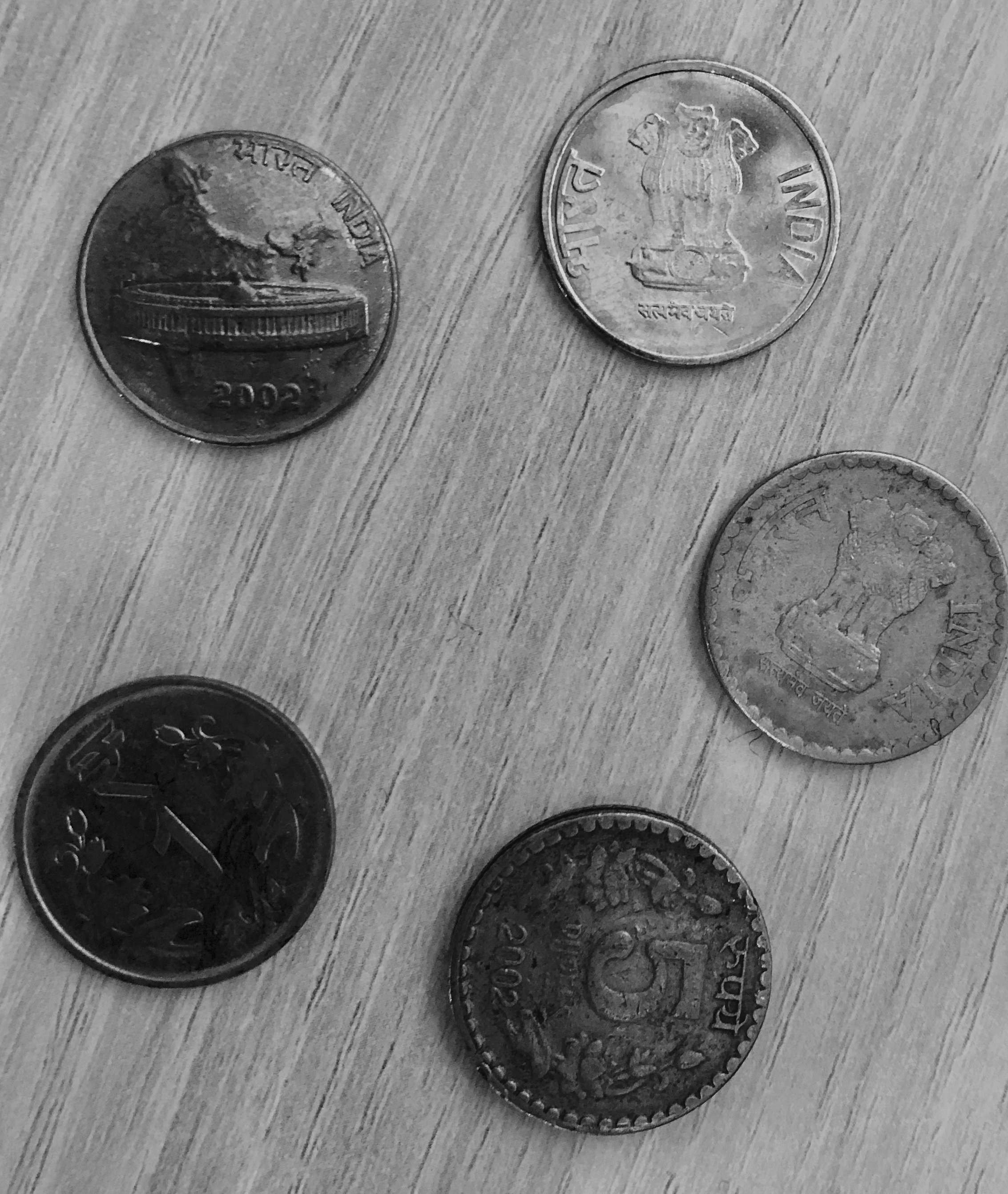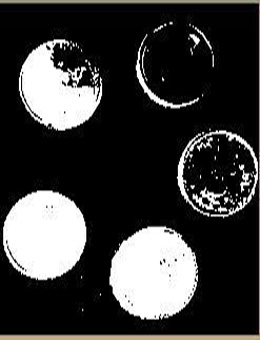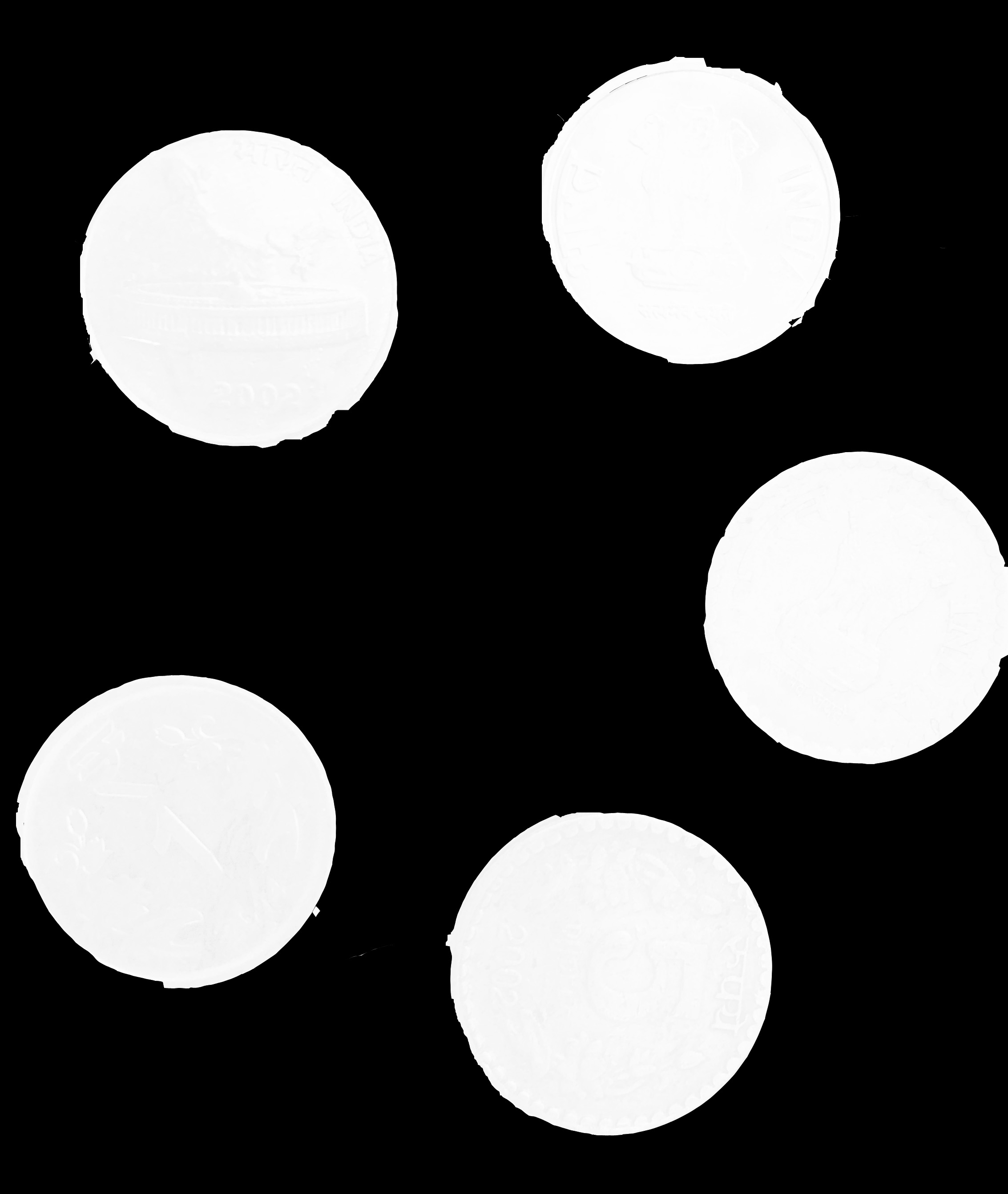# Image segmentation using Morphological operations in Python

If we want to extract or define something from the rest of the image, eg. detecting an object from a background, we can break the image up into segments in which we can do more processing on. This is typically called Segmentation.

Morphological operations are some simple operations based on the image shape. It is normally performed on binary images. Two basic morphological operators are Erosion and Dilation. For basic understanding about Dilation and Erosion, refer this article.

In order to process on we’ll use OTSU’s threshold algorithm where this removes over segmented result due to noise or any other irregularities in the image and implement with OpenCV.

Approach :

• Label the region which we are sure of being the foreground or object with one color (or intensity), Label the region which we are sure of being background or non-object with another color.
• Finally the region which we are not sure of anything, label it with 0. That is our marker. Then apply watershed algorithm.
• Then our marker will be updated with the labels we gave, and the boundaries of objects will have a value of -1.

Input Image :`# Python program to transform an image using ` `# threshold. ` `import` `numpy as np ` `import` `cv2 ` `from` `matplotlib ``import` `pyplot as plt ` ` `  `# Image operation using thresholding ` `img ``=` `cv2.imread(``'c4.jpg'``) ` ` `  `gray ``=` `cv2.cvtColor(img, cv2.COLOR_BGR2GRAY) ` ` `  `ret, thresh ``=` `cv2.threshold(gray, ``0``, ``255``, ` `                            ``cv2.THRESH_BINARY_INV ``+` `                            ``cv2.THRESH_OTSU) ` `cv2.imshow(``'image'``, thresh) `

Output :This output shows that image is transformed by thresholding operation where foreground still contains some noises.

Now, we need to remove any small white noises in the image i.e. foreground. For that we can use morphological closing. To remove any small holes in the foreground object, we can use morphological closing. To obtain background we dilate the image. Dilation increases object boundary to background.

Let’s see the code :

 `# Noise removal using Morphological ` `# closing operation ` `kernel ``=` `np.ones((``3``, ``3``), np.uint8) ` `closing ``=` `cv2.morphologyEx(thresh, cv2.MORPH_CLOSE, ` `                            ``kernel, iterations ``=` `2``) ` ` `  `# Background area using Dialation ` `bg ``=` `cv2.dilate(closing, kernel, iterations ``=` `1``) ` ` `  `# Finding foreground area ` `dist_transform ``=` `cv2.distanceTransform(closing, cv2.DIST_L2, ``0``) ` `ret, fg ``=` `cv2.threshold(dist_transform, ``0.02` `                        ``*` `dist_transform.``max``(), ``255``, ``0``) ` ` `  `cv2.imshow(``'image'``, fg) `

Output :From the output image we can conclude that small holes are removed using closing operation and make sure whatever region in foreground in result is really a foreground.

My Personal Notes arrow_drop_upCheck out this Author's contributed articles.

If you like GeeksforGeeks and would like to contribute, you can also write an article using contribute.geeksforgeeks.org or mail your article to contribute@geeksforgeeks.org. See your article appearing on the GeeksforGeeks main page and help other Geeks.

Please Improve this article if you find anything incorrect by clicking on the "Improve Article" button below.

Article Tags :

1

Please write to us at contribute@geeksforgeeks.org to report any issue with the above content.